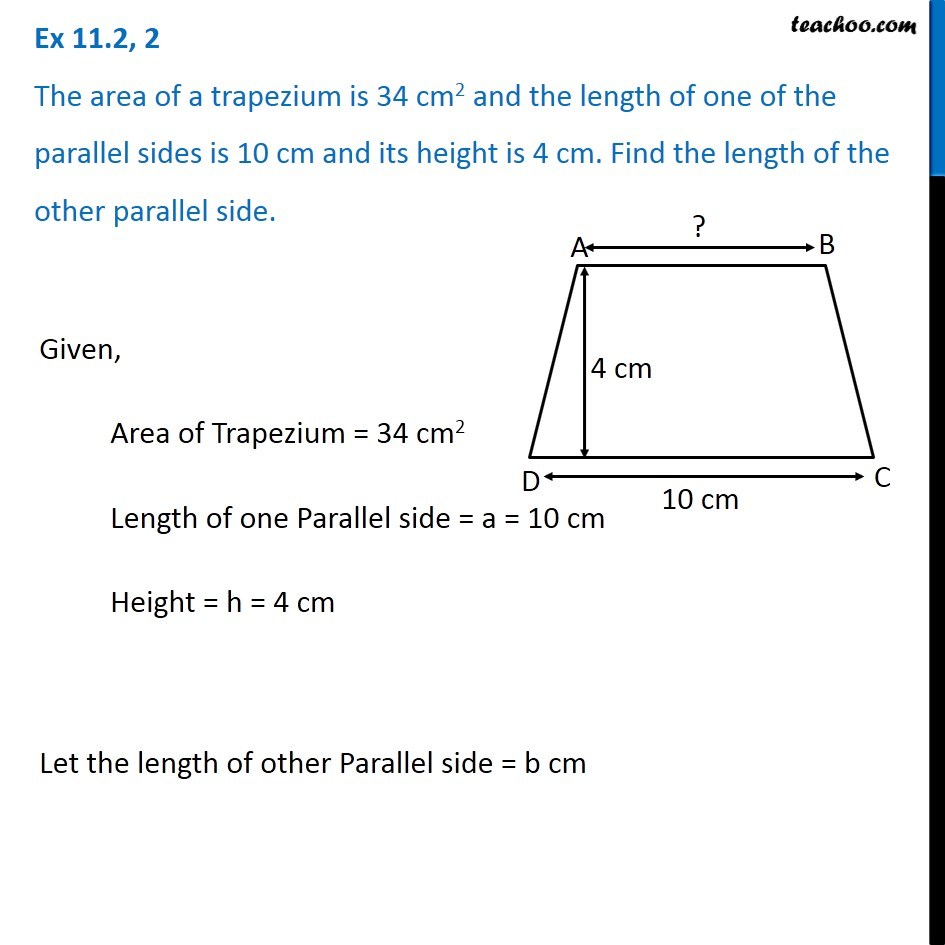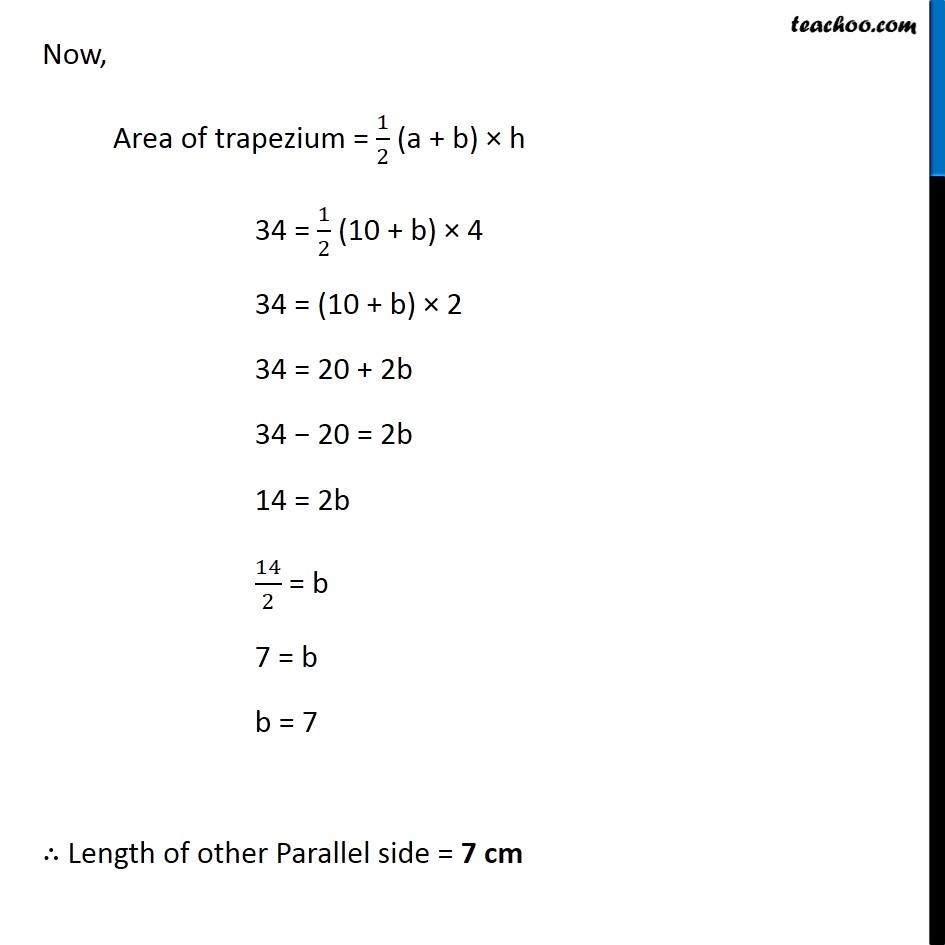Subscribe to our Youtube Channel - https://you.tube/teachoo

1. Chapter 11 Class 8 Mensuration
2. Concept wise
3. Area of Trapezium

Transcript

Ex 11.2, 2 The area of a trapezium is 34 cm2 and the length of one of the parallel sides is 10 cm and its height is 4 cm. Find the length of the other parallel side. Given, Area of Trapezium = 34 cm2 Length of one Parallel side = a = 10 cm Height = h = 4 cm Let the length of other Parallel side = b cm Now, Area of trapezium = 1/2 (a + b) × h 34 = 1/2 (10 + b) × 4 34 = (10 + b) × 2 34 = 20 + 2b 34 − 20 = 2b 14 = 2b 14/2 = b 7 = b b = 7 ∴ Length of other Parallel side = 7 cm

Area of Trapezium